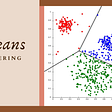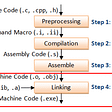# Understanding Bidirectional Dijkstra’s Algorithm

Dijkstra’s algorithm is well known shortest path finding algorithm in a graph. But there exists Bidirectional Dijkstra algorithm as well which runs two simultaneous searches, one in the forward direction from source and the other in the backward direction from destination until they meet eventually. In this article, we will cover the algorithm with an example and implement it in Java Language.

# Introduction

The algorithm appears to be complex at the beginning but once you get the picture it’s quite simple actually. Assume the graph G with vertices V and edges E. Also, imagine that there is another Graph G’ which has all the edges in G reversed. Now let’s start the search from source vertex G and destination vertex in G’ simultaneously till the search spaces meet. With this approach, search space is reduced to nearly a factor of 2.

# Algorithm

• Like Dijkstra’s algorithm, start search from source vertex u in G and destination vertex v in G’
• Maintain DistanceF,DistanceB map for storing min distances of vertices from u and v respectively initialised to
• Lets maintain priority Queues QueueF for forward and QueueB for backward searches
• Let there be a Join Node which are vertices found common in forward and backward searches.
• Let there be µ (Estimated or Best Path seen so far).
• Push the u and v to QueueF and QueueB respectively.
• Check min in QueueF,QueueB , Let vertex be x, and adjacent vertex be y , perform forward and backward search. Also, update µ if DistanceF (x) + Weight of Edge(x, y) + DistanceB(y) < µ
• Stopping the search when QueueF.top.distance + QueueB.top.distance >= µ

Lets understand the algorithm using following example:

As we can see in this example the shortest path from source”a” to destination “e” is [ a, h, g, f, e] with distance 21. Lets try to run Bidirectional Dijkstra’s algorithm on above graph to find shortest path from “a” to “e”

• The table associated with each diagram represents min distances from source vertex (found so far) in forward search (F) and destination vertex in backward search (B).
• Let QF, QB represents QueueF and QueueB which are used in forward and backward search respectively. These are priority queues represented as [distance, vertex] with min distance vertex on the left side. e.g. [4,b] in QF represents distance of vertex b from source vertex a is 4.

Let’s understand the algorithm with steps.

• Step 1, add source and destination vertex to forward and backward priority queues.
• Step 2, Poll min element from QB (in this case ‘e’) and append ‘d’ and ‘f’ to the queue.
• Step 3, Since QF has min distance [0,a] lets poll the element from queue and add ‘b’ and ‘h’ to queue
• Step 4, Again, Since QF has min distance [4, b] let’s poll it and add ‘c’ to the queue.
• Step 5, Again, Since QF has min distance [8, h] let’s poll it and add ‘g’ and ‘i’ to the queue.
• Step 6, Now since both QF and QB has same value, let’s poll [9,d] from QB. We have found the first Join Node ‘c’ and the path from source to destination is [a, b, c, d, e] with distance µ=28. But, obviously it is not the shortest path.
• Step 7, min value is in QF [9,g] let’s poll it. We have found the second Join Node ‘f’ with µ=21 which is less than 28 and the shortest path is [a, h, g, f, e] with distance 21.
• Lets check if QueueF.top.distance + QueueB.top.distance >= µ, which is 12+10>=21, we stop.

We can see that we have found shortest path [a, h, g, f, e] with distance 21 using bidirectional dijkstra’s algorithm.

# Implementation

Here is the Java Implementation for Bidirectional Dijkstra’s Algorithm which computes both minimum distance and path from source to destination:

# Final Thoughts

Graphs can be mapped to various real-world situations as there are road networks, computer networks, social networks, etc. We need faster search algorithms, sometimes correctness is more important than performance if compared to other algorithms like A*. Bidirectional Dijkstra is an important inspiration to many sophisticated algorithms like Contraction Hierarchies.

# References

--

--

--

## More from Ritesh Kapoor

Software Engineer — Passionate about Algorithms, Architectural Designs, Agile Methodologies

Love podcasts or audiobooks? Learn on the go with our new app.

## 10 Commandments Leading Our DevOps Journey in Kongsberg Digital## How to measure the similarity between two strings with Dart## Seeing code in the config## Vision AI and 8 images similar to yours## Make Way For The Matrix — A Complete Guide to Solving 2D Array Coding Problems## CTF — hacker101 — Photo Gallery## A DynamoDB-backed CRUDL example using Golang## Activerecord Rails to Ecto Elixir## Ritesh Kapoor

Software Engineer — Passionate about Algorithms, Architectural Designs, Agile Methodologies

## k-means clustering and its real use case in the security domain:## ¿What is a static library in C and how to use them?## Divine providence vs the algorithm## How to be a part of an NGO?